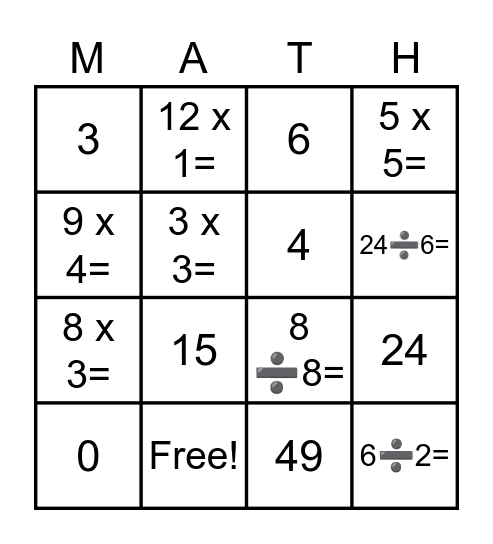# Multiplication and Division BingoThis bingo card has 31 words: 9, 25, 24, 12, 3, 6, 4, 5, Free!, 36, 49, 0, 21, 1, 15, 45, 7 x 3=, 50➗10=, 6➗2=, 1 x 0=, 9 x 4=, 30➗2=, 7 x 7=, 12 x 1=, 8 x 3=, 3 x 3=, 5 x 5=, 8 ➗8=, 5 x 9=, 24➗6= and 30➗5=.

## Play Online